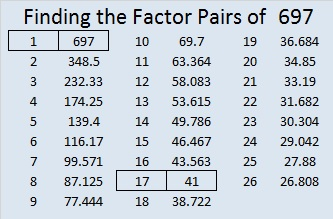# 697 and Level 3

• 697 is a composite number.
• Prime factorization: 697 = 17 x 41
• The exponents in the prime factorization are 1 and 1. Adding one to each and multiplying we get (1 + 1)(1 + 1) = 2 x 2 = 4. Therefore 697 has exactly 4 factors.
• Factors of 697: 1, 17, 41, 697
• Factor pairs: 697 = 1 x 697 or 17 x 41
• 697 has no square factors that allow its square root to be simplified. √697 ≈ 26.40075756.Here is today’s factoring puzzle:Print the puzzles or type the solution on this excel file: 12 Factors 2015-11-30

———————————————————————————

Here are some more thoughts about the number 697:

Because both of its factors are hypotenuses of Pythagorean triples, 697 is the hypotenuse of FOUR Pythagorean triples:

• 153-680-697
• 185-672-697
• 328-615-697
• 455-528-697

Two of those triples are primitive, and two are not. Can you find the greatest common factor for each one that is not primitive?

697 is palindrome 151 in BASE 24; note that 24² = 576, and 1(576) + 5(24) + 1(1) = 697.

———————————————————————————

A Logical Approach to solve a FIND THE FACTORS puzzle: Find the column or row with two clues and find their common factor. (None of the factors are greater than 12.)  Write the corresponding factors in the factor column (1st column) and factor row (top row).  Because this is a level three puzzle, you have now written a factor at the top of the factor column. Continue to work from the top of the factor column to the bottom, finding factors and filling in the factor column and the factor row one cell at a time as you go.This site uses Akismet to reduce spam. Learn how your comment data is processed.